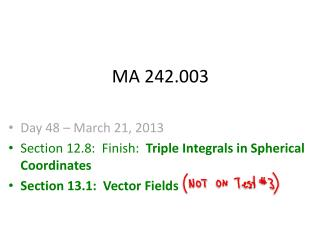DownloadDownload PresentationMA 242.003

# MA 242.003

Télécharger la présentation## MA 242.003

- - - - - - - - - - - - - - - - - - - - - - - - - - - E N D - - - - - - - - - - - - - - - - - - - - - - - - - - -
##### Presentation Transcript

1. MA 242.003 • Day 48 – March 21, 2013 • Section 12.8: Finish: Triple Integrals in Spherical Coordinates • Section 13.1: Vector Fields

2. Section 12.8 Triple Integrals in Cylindrical Coordinates Goal: Use spherical coordinates to compute a triple integral that has spherical symmetry. Spheres Cones

3. Fubini’s theorem for a spherical box:

4. Generalization to non-spherical boxes

5. Generalization to non-spherical boxes

6. Generalization to non-spherical boxes

7. (Continuation of example)

8. Chapter 13: Vector Calculus

9. Chapter 13: Vector Calculus “In this chapter we study the calculus of vector fields,

10. Chapter 13: Vector Calculus “In this chapter we study the calculus of vector fields, and line integrals of vector fields (work),

11. Chapter 13: Vector Calculus “In this chapter we study the calculus of vector fields, …and line integrals of vector fields (work), …and the theorems of Stokes and Gauss,

12. Chapter 13: Vector Calculus “In this chapter we study the calculus of vector fields, …and line integrals of vector fields (work), …and the theorems of Stokes and Gauss, …and more”

13. Section 13.1: Vector Fields

14. Section 13.1: Vector Fields

15. Section 13.1: Vector Fields Wind velocity vector field 2/20/2007

16. Section 13.1: Vector Fields Wind velocity vector field 2/21/2007 Wind velocity vector field 2/20/2007

17. Section 13.1: Vector Fields Ocean currents off Nova Scotia

18. Section 13.1: Vector Fields Airflow over an inclined airfoil.

19. General form of a 2-dimensional vector field

20. General form of a 2-dimensional vector field

21. General form of a 2-dimensional vector field

22. General form of a 2-dimensional vector field

23. General form of a 2-dimensional vector field Examples: QUESTION: How can we visualize 2-dimensional vector fields?

24. General form of a 2-dimensional vector field Examples: Question: How can we visualize 2-dimensional vector fields? Answer: Draw a few representative vectors.

25. Example:

26. Example: